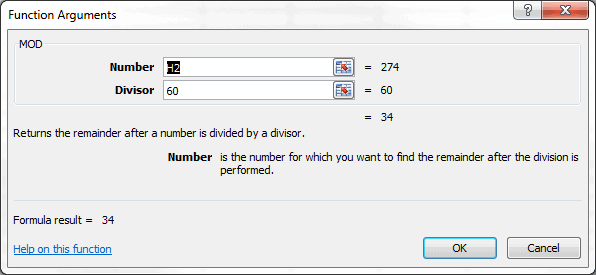# The MOD Function in Excel

The Excel MOD Function simply returns a remainder, or fractional part, after a number has been divided. To understand the MOD Function better, a look at how the INT Function can be used may provide some insight.

First, the calculation example is 274 seconds converted to 4:34.  The INT Function was used in a previous post, so we know that INT(274/60) = 4.

Since 4 minutes equals 240 seconds, the following three equations are true.

274 – 240 = 34

274 – 60 x 4 = 34

274 – 60 * INT(274/60) = 34

This is essentially the MOD Function, which has two arguments: Number and Divisor.

If n = 274, and d = 60, then the MOD Function is:

MOD(n,d) = n – d*INT(n/d)

Thinking about this a different way, 274/60 = 4.5667. If I subtract 4 from 4.5667, I get the fractional part 0.5667, which I can multiply by 60 to get the number of seconds: 34.

## Excel MOD Function

So the MOD Function is going to give the remainder. In this case the number of fractional seconds left over after dividing by 60. In my example:

MOD(274,60) = 34The next post will look at the TIME Function.## 2 thoughts on “The MOD Function in Excel”

1.michelle.simms

I am trying to convert minutes into seconds. Need to know the formula for that.
1.Gregory